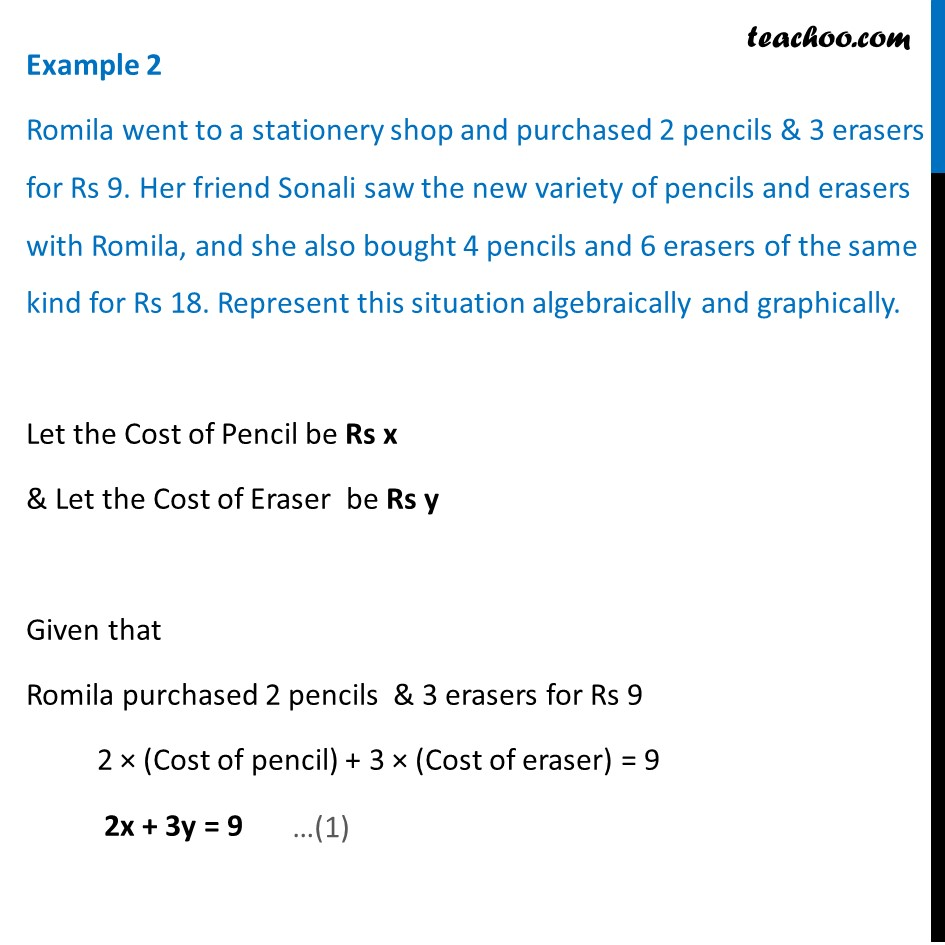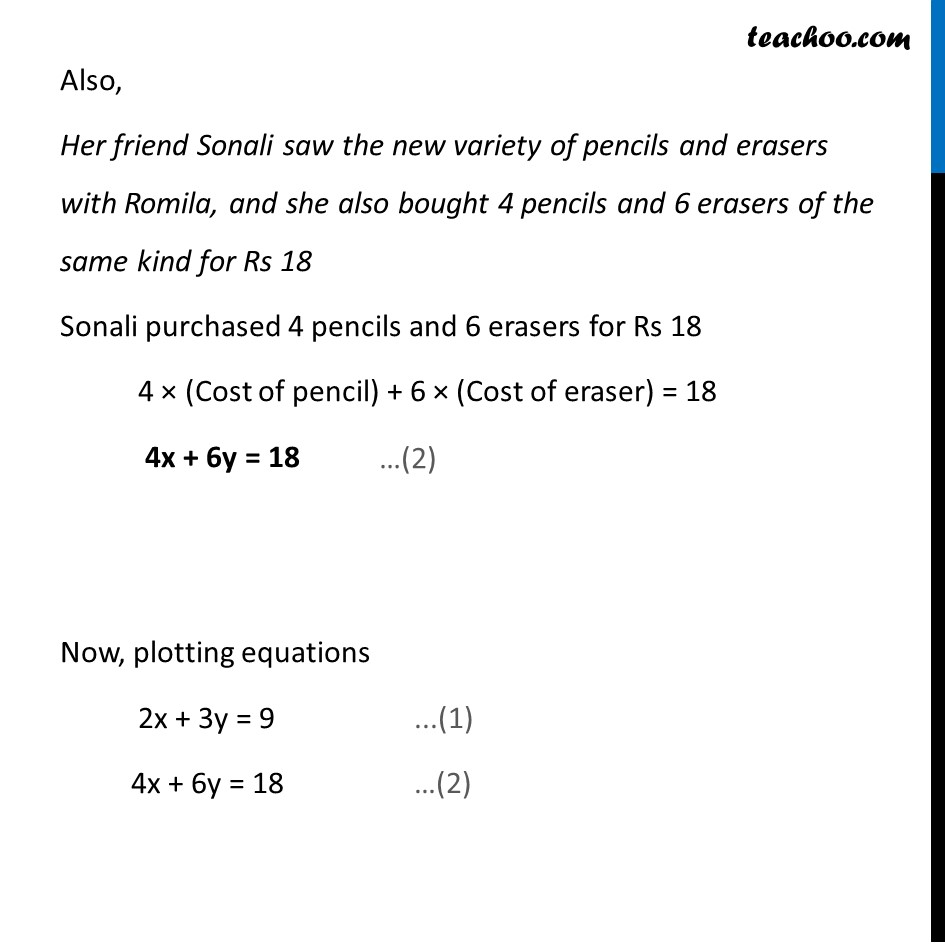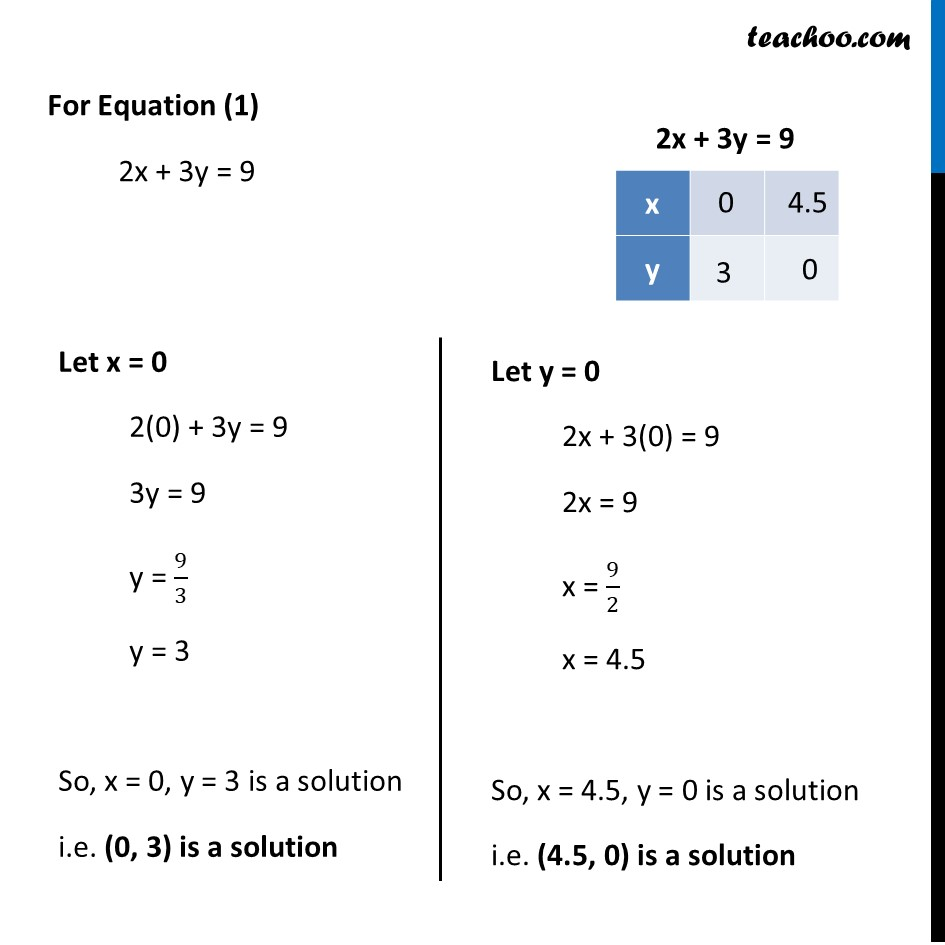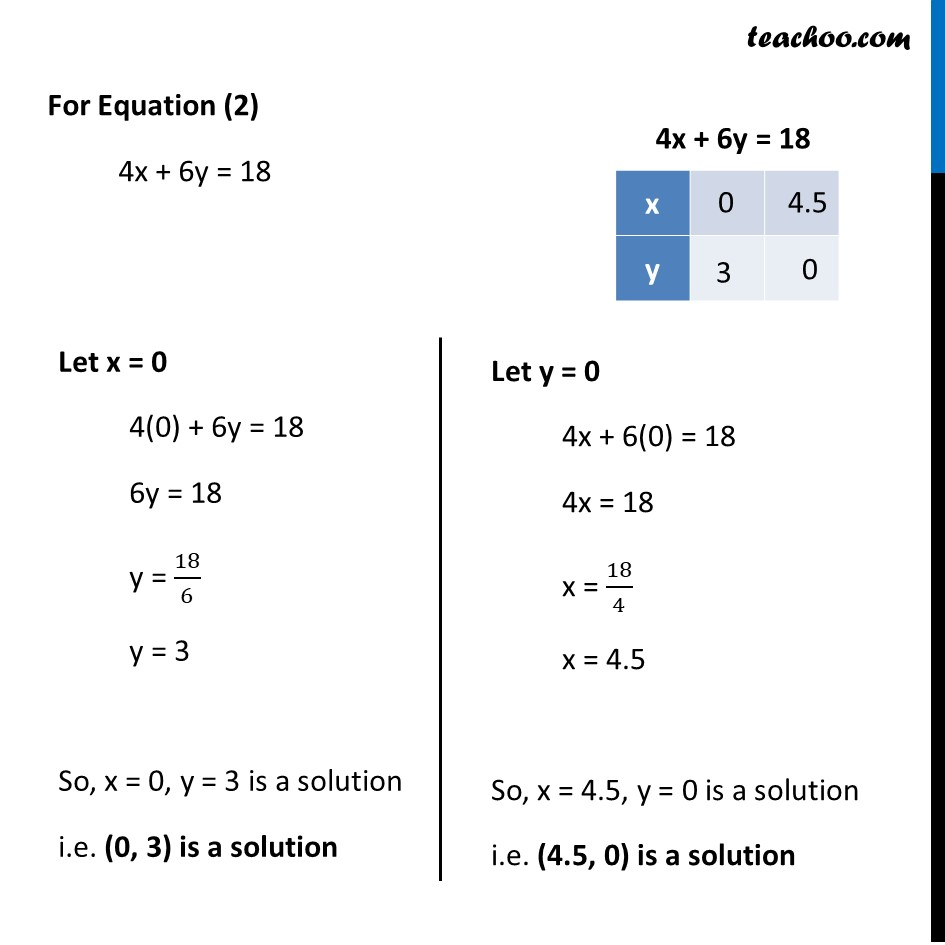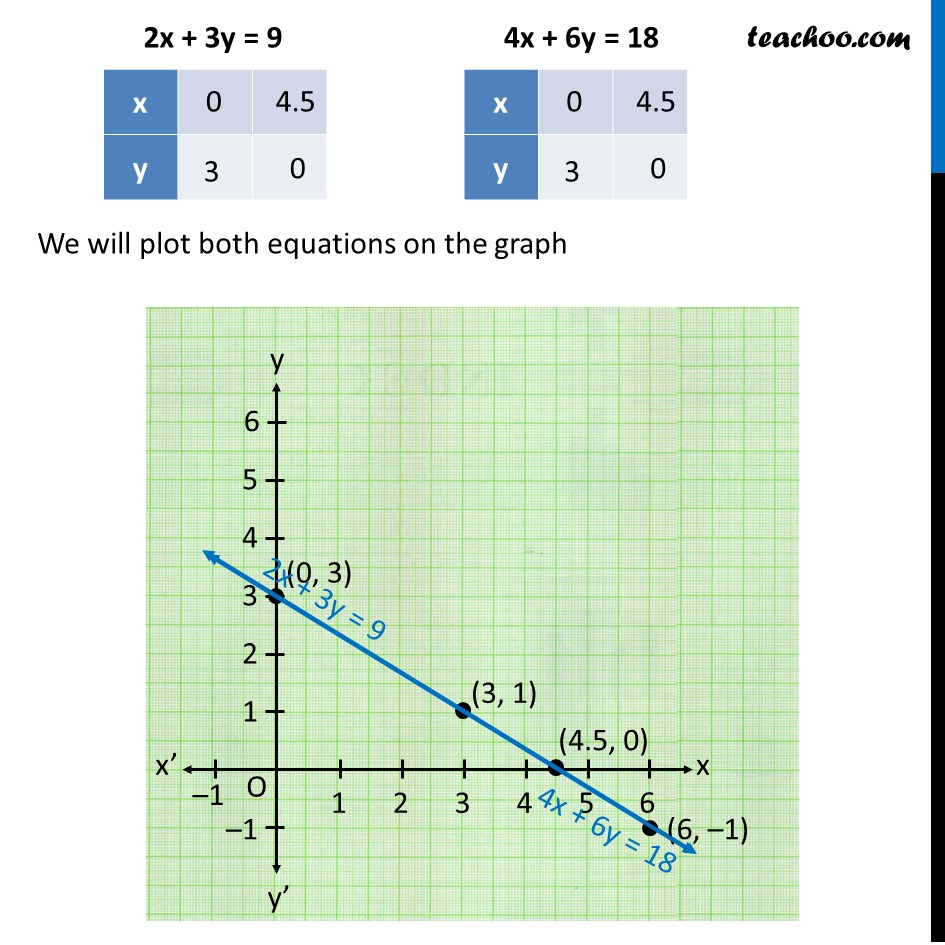1. Chapter 3 Class 10 Pair of Linear Equations in Two Variables (Term 1)
2. Concept wise
3. Forming equations graphically and algebraically

Transcript

Example 2 Romila went to a stationery shop and purchased 2 pencils & 3 erasers for Rs 9. Her friend Sonali saw the new variety of pencils and erasers with Romila, and she also bought 4 pencils and 6 erasers of the same kind for Rs 18. Represent this situation algebraically and graphically. Let the Cost of Pencil be Rs x & Let the Cost of Eraser be Rs y Given that Romila purchased 2 pencils & 3 erasers for Rs 9 2 × (Cost of pencil) + 3 × (Cost of eraser) = 9 2x + 3y = 9 Also, Her friend Sonali saw the new variety of pencils and erasers with Romila, and she also bought 4 pencils and 6 erasers of the same kind for Rs 18 Sonali purchased 4 pencils and 6 erasers for Rs 18 4 × (Cost of pencil) + 6 × (Cost of eraser) = 18 4x + 6y = 18 Now, plotting equations 2x + 3y = 9 ...(1) 4x + 6y = 18 …(2) For Equation (1) 2x + 3y = 9 Let x = 0 2(0) + 3y = 9 3y = 9 y = 9/3 y = 3 So, x = 0, y = 3 is a solution i.e. (0, 3) is a solution Let y = 0 2x + 3(0) = 9 2x = 9 x = 9/2 x = 4.5 So, x = 4.5, y = 0 is a solution i.e. (4.5, 0) is a solution For Equation (2) 4x + 6y = 18 Let x = 0 4(0) + 6y = 18 6y = 18 y = 18/6 y = 3 So, x = 0, y = 3 is a solution i.e. (0, 3) is a solution Let y = 0 4x + 6(0) = 18 4x = 18 x = 18/4 x = 4.5 So, x = 4.5, y = 0 is a solution i.e. (4.5, 0) is a solution We will plot both equations on the graph